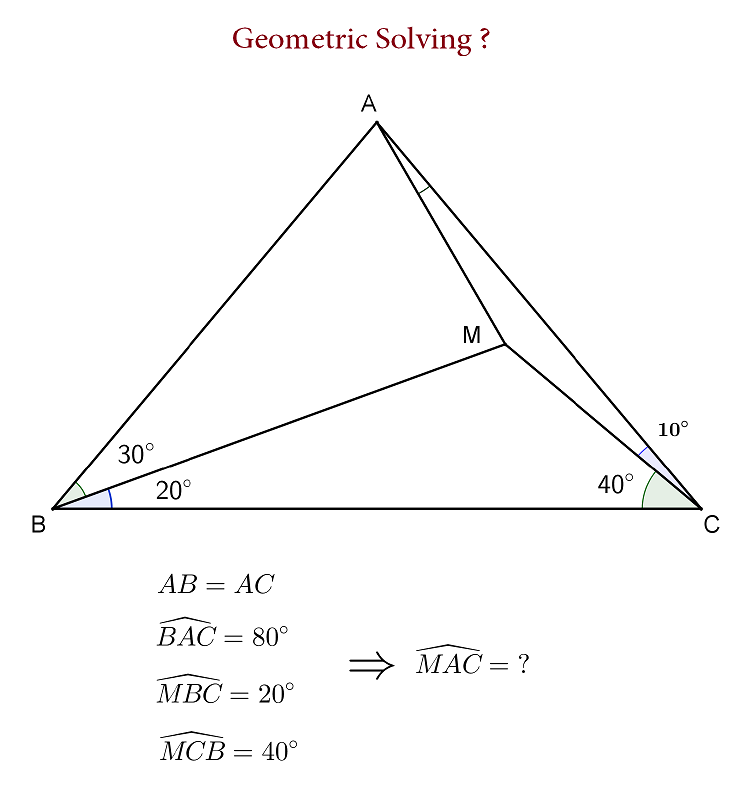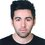# Geometric solution

I found a trigonometric solution to the following problem:Can you find a solution without using trigonometry?Note by Kazem Sepehrinia
2 years, 8 months ago

This discussion board is a place to discuss our Daily Challenges and the math and science related to those challenges. Explanations are more than just a solution — they should explain the steps and thinking strategies that you used to obtain the solution. Comments should further the discussion of math and science.

When posting on Brilliant:

• Use the emojis to react to an explanation, whether you're congratulating a job well done , or just really confused .
• Ask specific questions about the challenge or the steps in somebody's explanation. Well-posed questions can add a lot to the discussion, but posting "I don't understand!" doesn't help anyone.
• Try to contribute something new to the discussion, whether it is an extension, generalization or other idea related to the challenge.

MarkdownAppears as
*italics* or _italics_ italics
**bold** or __bold__ bold
- bulleted- list
• bulleted
• list
1. numbered2. list
1. numbered
2. list
Note: you must add a full line of space before and after lists for them to show up correctly
paragraph 1paragraph 2

paragraph 1

paragraph 2

[example link](https://brilliant.org)example link
> This is a quote
This is a quote
    # I indented these lines
# 4 spaces, and now they show
# up as a code block.

print "hello world"
# I indented these lines
# 4 spaces, and now they show
# up as a code block.

print "hello world"
MathAppears as
Remember to wrap math in $$ ... $$ or $ ... $ to ensure proper formatting.
2 \times 3 $2 \times 3$
2^{34} $2^{34}$
a_{i-1} $a_{i-1}$
\frac{2}{3} $\frac{2}{3}$
\sqrt{2} $\sqrt{2}$
\sum_{i=1}^3 $\sum_{i=1}^3$
\sin \theta $\sin \theta$
\boxed{123} $\boxed{123}$

Sort by:

Redefine point $A$ to satisfy $MA=MC$ and $\angle MAC= \angle MCA=10^{\circ}.$ So, now it suffices to prove that $\angle ABM=30^{\circ}$ or $AB=AC.$ Let $D$ be a point (below $BC$) such that $MB=MD, \angle BMD= 40^{\circ},$ and $MD\cap BC\equiv E.$ Denote $I$ as the incenter of $\Delta MEC. F\in BM: ME=MF, G\in BC :FE=FG.$ Clearly, $\Delta MDA \cong \Delta MBC \Rightarrow \angle MDA=20^{\circ}, \angle MAD=40^{\circ}, \angle CAD=50^{\circ} --(1)$

We have; $\angle MFE=70^{\circ}$ and $\angle MIE= 90^{\circ}+\frac{\angle MCE}{2} = 110^{\circ} \Rightarrow$ quad. $MIEF$ is cyclic. Also, since $ME$ bisects $\angle IMF \Rightarrow IE=EF=GF$ Now, note that $\Delta BFG\sim \Delta CEI$ by $AAS-$ similarity $\Rightarrow BF=CE$ But due to symmetry in isosceles $\Delta BMD, BF=ED$ Combining the two we get, $CE=ED \Rightarrow \angle MDC= 30^{\circ} \therefore,$ from result $(1) \angle CDA=50^{\circ}=\angle CAD \Rightarrow CA=CD$ Also, $\Delta MCD\cong\Delta MAB \Longrightarrow AB=CD=AC$. And, so done. $\boxed{Q.E.D}$

- 2 years, 7 months ago

Beautiful solution, thanks :)

- 2 years, 3 months ago

Have you tried an algebraic solution to this, involving all the unknown angles?

P.S. All the methods used still fall under the banner of "trigonometry", as ultimately we are studying triangles.

- 2 years, 8 months ago

I used sin law several times and then a trigonometric equation comes out for the unknown MAC angle. Solving this equation gives MAC=10 degrees. But I wanted to use only basic geometric rules like parallel lines, isosceles triangles, sum of angles of a triangle. For example if we could prove AM=MC then problem is solved.

- 2 years, 8 months ago

You can solve it algebraically, as i said, in terms of the unknown angles, for which there are four. Fortunately, all the equations will be linear, so you will need to (hopefully) find four relations from the triangle that allow you to solve using linear algebraic methods.

To start you off, the angles around M add up to a full circle, so you can find a relation between two of the four unknown angles (as one of the angles is easily obtainable); this relation would namely be the sum.

- 2 years, 8 months ago

@Kazem Sepehrinia Well, I don't have a solution without trigonometry, but we can definitely solve it quickly by applying the Sine Rule to Triangles AMC and AMB and take advantage of the fact that AB = AC along with AM = AM (the common side).....!!

- 2 years, 8 months ago

There are four unknown angles (as the fifth can be easily obtained), and four possible relationships involving the unknown which turn out to be all linear in the unknowns. This must mean that a linear algebraic solution can easily be obtained.

- 2 years, 8 months ago

i found a solution with basic geometry and got the answer 10 ... is it correct?

- 2 years, 8 months ago

- 2 years, 8 months ago

- 2 years, 8 months ago

please draw step by step with me... extend AB to meet AC at D.Again extend MD to reach a point E which MD=DE.So as you can see AM=AE and triangle EBM is equilateral. Take a point P in triangle MEB such that angle PBA=10.Thus,two triangles ABP & AMC are congruent.So we got that AP=AM=AE and angle MAP=80 and PB=CM. Draw a circle with radius AM.As you can see angle PAM=2*PEM=80>>>>PEM=40>>>>>BEP=PBE=20>>>>>PB=PE. PB=BE PM=PM(common)>>>>>>>>>>>>>>>>>>>>triangles MPE & BPM are congruent>>>>>>>angles EMP=PMB=30>>>>>>>>angle PAE=60(in circle) BM=Em So triangle PEA is also equilateral>>>>>EP=AM=BP=CM. after all sorry for my English.... :)

- 2 years, 8 months ago

Nice natural approach:)) There is a bit typo in the first line, it should be "CM" rather than "AB".

- 2 years, 7 months ago

I have also a new method

- 2 years, 1 month ago

I have proved it without congruence

- 2 years, 1 month ago

try. this.. or **else . .. draw a perpendicular from a on bc.. and now. daw. the same figure symmetric abt.. AP then mark the point on AP where lines intersect as Q .. then.. produce. BM. till it meets AC .. and then also drop a perpendicular from M on AC name it N .repeat same... symmetrically. on othr side.. then.. use. basic geometry..(properties of isosceles triangle. and.. exterior angle theorem and. solve fr all the angles.. .. you will realise that. angle ANM is equal to angle AQM. equal to 70 degress. then use again properties of similar triangle.. n find. 2x=180 -2(70)=> x=20 this. is entirely. geometry

- 2 years, 7 months ago

Sorry, but the correct answer is 10 degrees.............

- 2 years, 7 months ago

ABC is a triangle with angle bisector AD,BE,and CF. If angle FDE = 90 degree then find the angle BAC?

- 2 years, 6 months ago

$120^{\circ}$ ---

- 2 years, 6 months ago

- 2 years, 6 months ago

How it is came? Send me the COMPLETE solution .In this way i would able to findmy mistake. Of Q1, ABC is a triangle with angle bisector AD,BE,and CF. If angle FDE = 90 degree then find the angle BAC

- 2 years, 5 months ago

I have solve it

- 2 years, 1 month ago

It is 10

- 2 years, 1 month ago

Compas, D Scale, Triangle Scale and many more types of equipment for geometry diagram. Here ( https://www.mistersaad.com/ )Mobile app developer create Geometry application with complete Guide.

- 1 year, 10 months ago

every good article. I am going through a few of these issues as well. Also, I am creating the latest trend websites you can visit my web site: https://www.adwebstudio.com/web-design-company-dubai

- 1 year, 10 months ago

Thanks a lot for this Geometric solution post. Keep up the good work. I’ll be coming back lots. I am working on a web design company and we create all kind of websites you can visit our website: https://www.adwebstudio.com/web-design-company-riyadh-jeddah-saudi-arabia

- 1 year, 10 months ago

take a protector.. scale compass.. draw thefig. and measure

- 2 years, 7 months ago